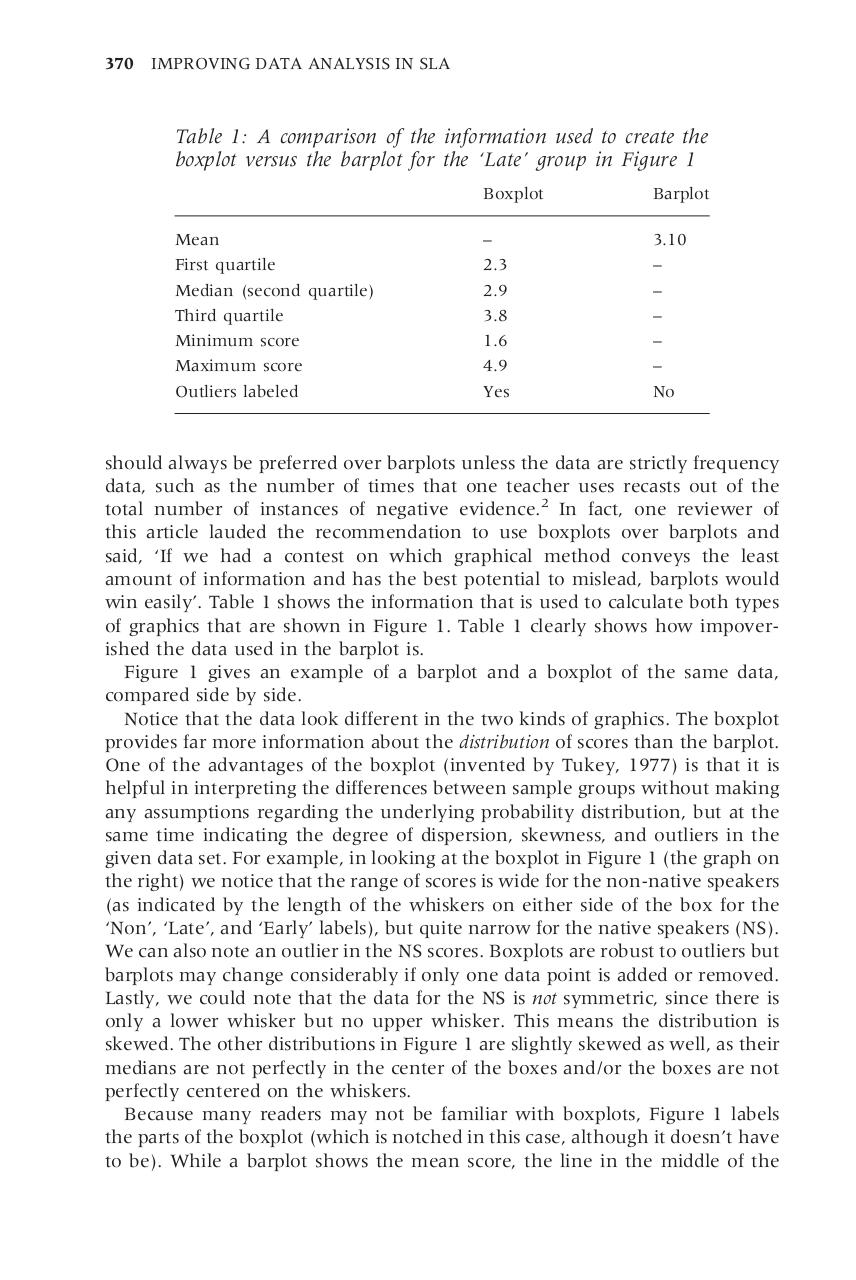10.pdfPage 1 2 34524

Text preview

370 IMPROVING DATA ANALYSIS IN SLA

Table 1: A comparison of the information used to create the
boxplot versus the barplot for the ‘Late’ group in Figure 1

Mean
First quartile
Median (second quartile)
Third quartile
Minimum score
Maximum score
Outliers labeled

Boxplot

Barplot

2.3
2.9
3.8
1.6
4.9
Yes

3.10

No

should always be preferred over barplots unless the data are strictly frequency
data, such as the number of times that one teacher uses recasts out of the
total number of instances of negative evidence.2 In fact, one reviewer of
said, ‘If we had a contest on which graphical method conveys the least
amount of information and has the best potential to mislead, barplots would
win easily’. Table 1 shows the information that is used to calculate both types
of graphics that are shown in Figure 1. Table 1 clearly shows how impoverished the data used in the barplot is.
Figure 1 gives an example of a barplot and a boxplot of the same data,
compared side by side.
Notice that the data look different in the two kinds of graphics. The boxplot
One of the advantages of the boxplot (invented by Tukey, 1977) is that it is
helpful in interpreting the differences between sample groups without making
any assumptions regarding the underlying probability distribution, but at the
same time indicating the degree of dispersion, skewness, and outliers in the
given data set. For example, in looking at the boxplot in Figure 1 (the graph on
the right) we notice that the range of scores is wide for the non-native speakers
(as indicated by the length of the whiskers on either side of the box for the
‘Non’, ‘Late’, and ‘Early’ labels), but quite narrow for the native speakers (NS).
We can also note an outlier in the NS scores. Boxplots are robust to outliers but
barplots may change considerably if only one data point is added or removed.
Lastly, we could note that the data for the NS is not symmetric, since there is
only a lower whisker but no upper whisker. This means the distribution is
skewed. The other distributions in Figure 1 are slightly skewed as well, as their
medians are not perfectly in the center of the boxes and/or the boxes are not
perfectly centered on the whiskers.
Because many readers may not be familiar with boxplots, Figure 1 labels
the parts of the boxplot (which is notched in this case, although it doesn’t have
to be). While a barplot shows the mean score, the line in the middle of the